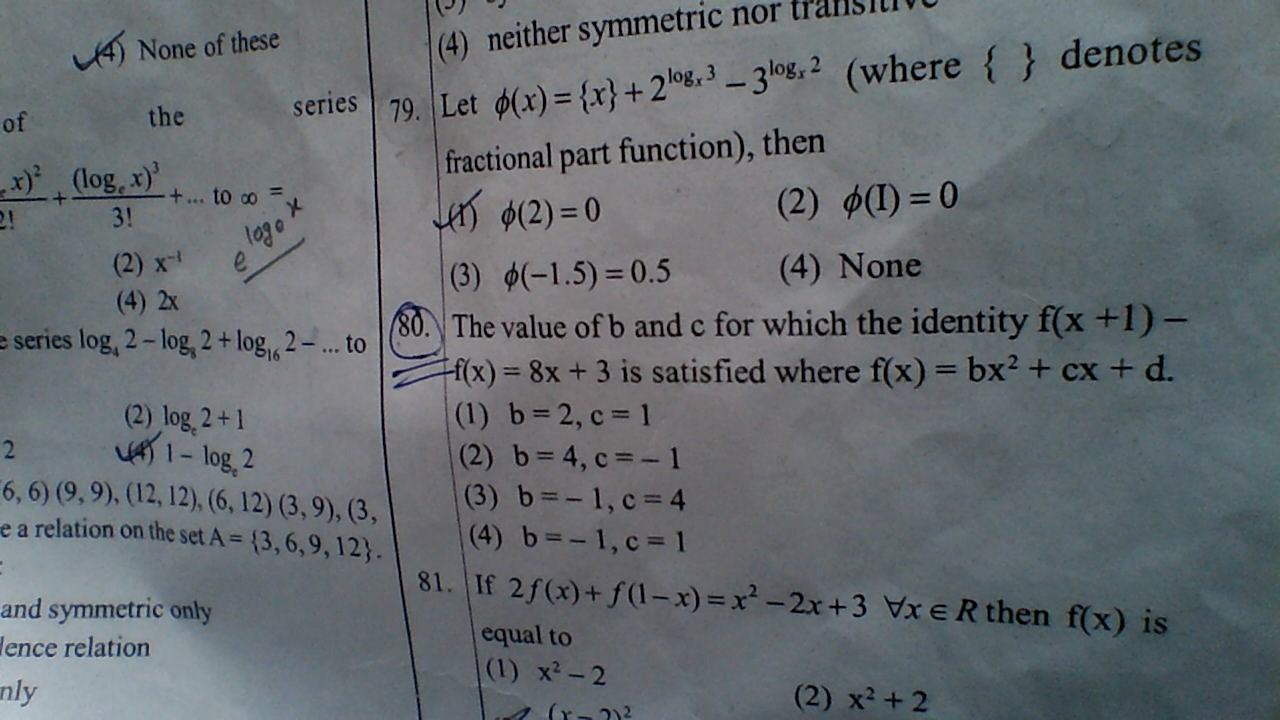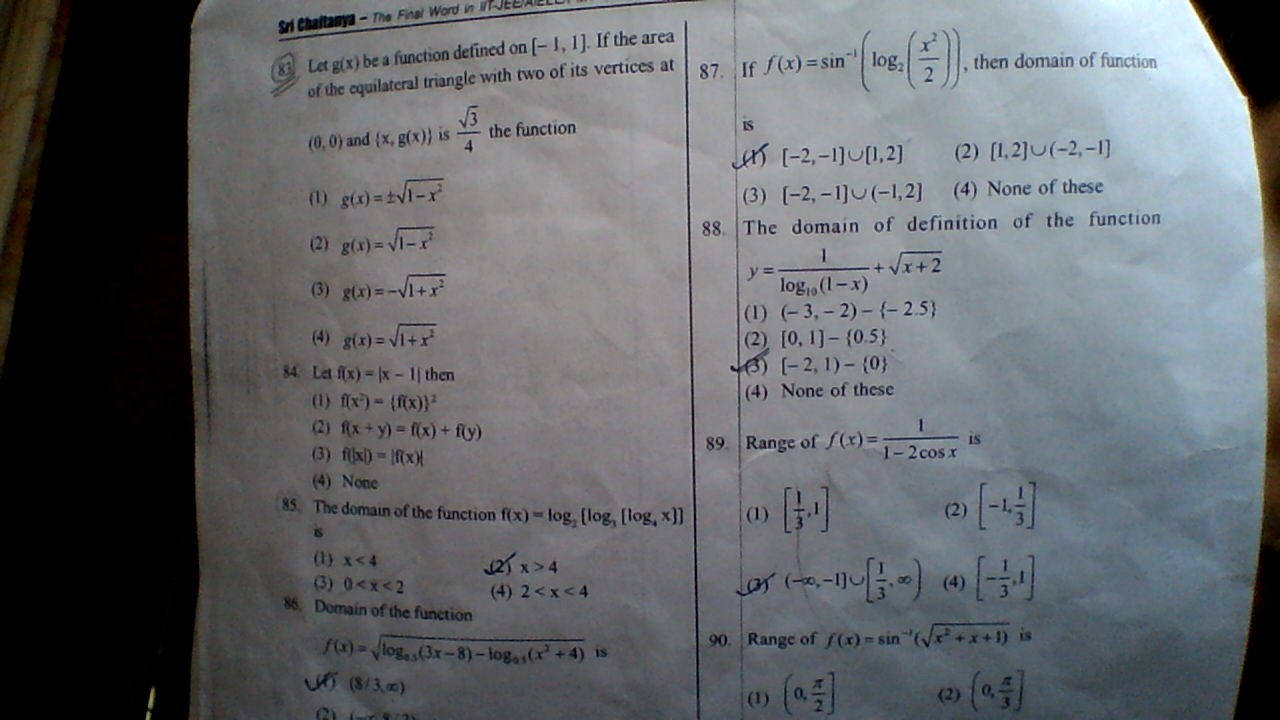# functions(q.80)•\hspace{-20}$Given$\bf{f(x+1)-f(x)=8x+3.......................(1)}$\\\\\\ and$\bf{f(x)=bx^2+cx+d}$\\\\\\ Replace$\bf{x\rightarrow (x+1)\;,}$we get$\bf{f(x+1)=b(x+1)^2+c(x+1)+d}$\\\\\\ Put into Equation....$\bf(1)$\\\\\\$\bf{\Rightarrow \left\{b(x+1)^2+c(x+1)+d\right\}-\left\{bx^2+cx+d\right\}=8x+3}$\\\\\\$\bf{\Rightarrow b(2x+1)+c = 8x+3}$\\\\\\ Now Camparing Coefficients, we get.......\\\\\\$\bf{\Rightarrow b=4}$and$\bf{c=-1}$•shivendu gupta ·Amartya Kumar Mistry · for the q.83 simply apply distance formula to get the side of the triangle then apply the formula of area of triangle which would give answer as g(x)=±√1-x^2 •Amartya Kumar Mistry · my mistake it should be the area of the equilateral triangle •Amartya Kumar Mistry · simply apply the condition for modulus in sign-scheme.i think the answer is possibly D •man111 singh · \hspace{-20}(81)\;\;$Given $\bf{2f(x)+f(1-x) = x^2-2x+3............(1)}$\\\\\\ Here $\bf{R.H.S}$ is a Polynomial, So $\bf{L.H.S}$ must be polynomial...\\\\\\ So Here $\bf{f(x)}$ must be in the form of $\bf{(2)\;} Degree.$\\\\\\So Let $\bf{f(x)=ax^2+bx+c}$ and $\bf{f(1-x)=a(1-x)^2+b(1-x)+c}$\\\\\\ Put into $\bf{(1)\;,}$ we get\\\\\\ $\bf{\Rightarrow 2ax^2+2bx+2c+a(1-x)^2+b(1-x)+c = x^2-2x+3}$\\\\\\ Now camparing The Coefficients, we get\\\\\\ $\bf{\Rightarrow a= \frac{1}{3}\;,b=-\frac{4}{3}\;\;,c=\frac{4}{3}}$\\\\\\ So $\bf{f(x)=\frac{1}{3}\left\{x^2-4x+4\right\}=\frac{1}{3}\cdot\left(x-2\right)^2}$
•